# Maximal correlation coefficient

(diff) ← Older revision | Latest revision (diff) | Newer revision → (diff)
A measure of dependence of two random variablesand, defined as the least upper bound of the values of the correlation coefficients between the real random variablesand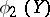, which are functions ofandsuch that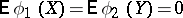and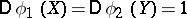: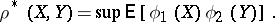If this least upper bound is attained at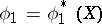and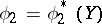, then the maximal correlation coefficient betweenandis equal to the correlation coefficient of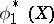and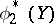. The maximal correlation coefficient has the property: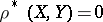is necessary and sufficient for the independence ofand. If there is a linear correlation between the variables, then the maximal correlation coefficient coincides with the usual correlation coefficient.